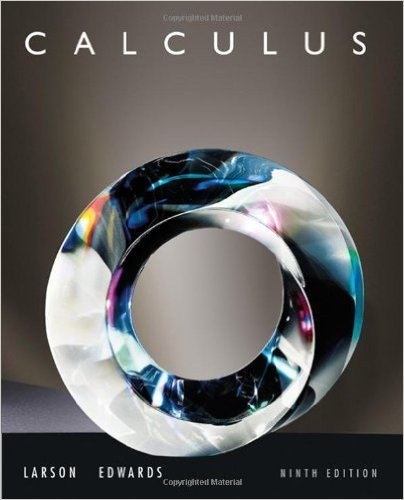×
×

# Solutions for Chapter 4.3: Riemann Sums and Definite Integrals## Full solutions for Calculus | 9th Edition

ISBN: 9780547167022Solutions for Chapter 4.3: Riemann Sums and Definite Integrals

Solutions for Chapter 4.3
4 5 0 341 Reviews
24
2
##### ISBN: 9780547167022

Since 80 problems in chapter 4.3: Riemann Sums and Definite Integrals have been answered, more than 61533 students have viewed full step-by-step solutions from this chapter. This textbook survival guide was created for the textbook: Calculus , edition: 9. This expansive textbook survival guide covers the following chapters and their solutions. Chapter 4.3: Riemann Sums and Definite Integrals includes 80 full step-by-step solutions. Calculus was written by and is associated to the ISBN: 9780547167022.

Key Calculus Terms and definitions covered in this textbook
• Absolute minimum

A value ƒ(c) is an absolute minimum value of ƒ if ƒ(c) ? ƒ(x)for all x in the domain of ƒ.

• Compounded monthly

See Compounded k times per year.

• Gaussian curve

See Normal curve.

• Half-angle identity

Identity involving a trigonometric function of u/2.

• Instantaneous rate of change

See Derivative at x = a.

• Inverse cosine function

The function y = cos-1 x

• Inverse function

The inverse relation of a one-to-one function.

• Linear equation in x

An equation that can be written in the form ax + b = 0, where a and b are real numbers and a Z 0

• Linear regression line

The line for which the sum of the squares of the residuals is the smallest possible

• n factorial

For any positive integer n, n factorial is n! = n.(n - 1) . (n - 2) .... .3.2.1; zero factorial is 0! = 1

• Normal distribution

A distribution of data shaped like the normal curve.

• Paraboloid of revolution

A surface generated by rotating a parabola about its line of symmetry.

• Polar equation

An equation in r and ?.

• Polynomial interpolation

The process of fitting a polynomial of degree n to (n + 1) points.

• Reciprocal identity

An identity that equates a trigonometric function with the reciprocal of another trigonometricfunction.

• Resolving a vector

Finding the horizontal and vertical components of a vector.

• Stemplot (or stem-and-leaf plot)

An arrangement of a numerical data set into a specific tabular format.

• Variable

A letter that represents an unspecified number.

• Vector

An ordered pair <a, b> of real numbers in the plane, or an ordered triple <a, b, c> of real numbers in space. A vector has both magnitude and direction.

• Vertex of an angle

See Angle.

×